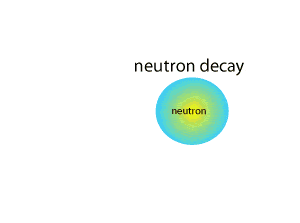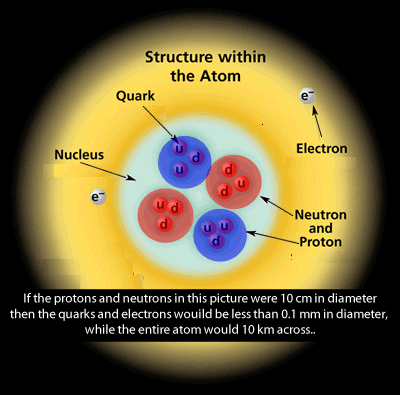Neutrino Neutrinos have no electrical charge and almost never interact with other particles. Most neutrinos pass through the earth without ever interacting with a single atom. One way neutrinos are produced is during a neutron decay. In fact, it was through a careful study of this type of radioactive decay that physicists hypothesized the neutrino's existence. In fact the prediction of the neutrino and its subsequent discovery is a fine example of how science works through observation, hypothesising investigating and predicting to build upon current understanding. In 1931 Wolfgang Pauli hypothesised the existence of the neutrino. After observations of certain radioactive decay he noticed that energy and momentum did not appear to be conserved. Newtons laws of conservation of energy and angular momentum, put forward over two centuries before, were not obeyed. Pauli suggested that this missing energy might be carried off, unseen, by a neutral particle which was escaping detection. In 1959 a particle fitting the expected characteristics of the neutrino was discovered. This neutrino is later determined to be the partner of the electron. Since then 2 other types of neutrinos have being discovered, the muon and the tau. Below is what Pauli observed. In a radioactive nucleus, a neutron at rest (zero momentum) decays, releasing a proton and an electron. Because of the law of conservation of momentum, the resulting products of the decay must have a total momentum of zero, which the observed proton and electron clearly do not. Therefore, we need to infer the presence of another particle with appropriate momentum to balance the event. Experiments have confirmed that this is indeed what happens.Once upon a time we thought that all matter was made from atoms which were composed of subatomic particles known as protons, neutrons and electrons. Now we know even these subatomic particles are made of smaller particles known as quarks, neutrinos leptons, just to name a few. The behaviour of these smaller particles is strange totally dissimilar to the behaviour of the larger subatomic particles such as the proton and electron. Just as an example, the force between two quarks becomes larger as they move farther apart, whereas the force between a proton and an electron, or two nucleons in a nucleus, grows weaker as their separation increases. Scientists have come up with the phrase the Standard Model to describe the current theory of fundamental particles and how they interact. The words model, hypothesis and theory are each used quite differently in science. Their use in science is also quite different than in everyday language. To scientists, the phrase "the theory of ..." signals a particularly well-tested idea. A hypothesis is a suggestion that has been put forward to explain a set of observations. It may be expressed in terms of a mathematical model. The model makes a number of predictions that can be tested in experiments. After many tests have been made, if the model can be refined to correctly describe the outcome of all experiments, it begins to have a greater status than a mere suggestion. Scientist do not use the term "the theory of .." except for those ideas that have been so thoroughly tested and developed that we know there is indeed some range of phenomena for which they give correct predictions every time. But, language being flexible, scientists may use "a theory" as a synonym for "a hypothesis". So, The Standard Model is a model and not a theory, as a theory is an idea that has been extensively tested. A Theory was orignally called a Law, but as sometimes theories aren't completely sound, it's best not to be as presumptious as to call it a Law. A model, is a series of theories that are put together to form a universal theory, in this case what Matter and Anti-Matter is made of. This text was adapted from http://www.phy.bris.ac.uk/groups/particle/PUS/A-level/Standard_Model.htm.We now have a better understanding of the many particles making up the atom and the universe. No longer do we view the proton, neutron as fundamental particles, although, the electron so far remains a fundamental particle. We now know that the proton and neutrons are made up of a number of particles such as quarks and gluons. Quarks are only found within protons and neutrons. Click to view the proton Click to view the neutron There are six types of quarks, of which the up and down quarks are found in protons and neutrons. Quarks are charged particles that have a charge of +2/3 or -1/3. There are also 6 types of leptons of which the electron is one of them. Leptons are said to be elementary particles; that is, they do not appear to be made up of smaller units of matter. Then there are the Force Carrier Particles which include the Photon, the Gluons, the Z boson and the W bosons. Gluons hold Quarks together in protons and neutrons. What is Newton's Law of conservation of angular momentum? What is Newton's Law of conservation of energy? How did Pauli use existing laws of physics to predict the existence of an unknown particle, the neutrino? When a neutron undergoes beta decay what are the particles that are produced? What is a fundamental particle? What is a proton composed of, in terms of fundamental particles? How does a neutron differ from a proton in its makeup? What are gluons? What is a hypothesis? What is a model? What is a theory? When does a theory become a law?